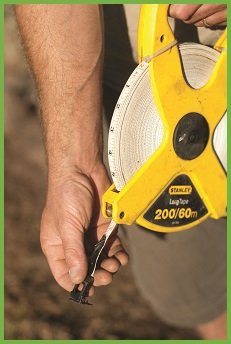# Installing Turf – Part 1 – MeasuringThe first step in installing turf needs to happen way before you even place the turf order – measuring. Using the Harden Park Lawns simple steps, calculating how many square metres of turf you need to order from us is a simple exercise.

How to calculate the amount of turf you will need

• Get a piece of paper and sketch out the areas where turf will be installed
• Divide up the areas into basic shapes such as squares, rectangles and circles.
• Measure each area and write down your measurements.
• Calculate how many square metres are in each area using the easy formulae list below.
• Add extra – for simple shape areas, order 5% extra and for more irregularly shaped areas, order 10% extra.
• Calculate the volume of turf underlay that you need.

## Working out the area of different shaped areas in your yard

Square
Measure one side of the square, then multiply it by itself.
Example – If the width of the square is 5 metres: 5m x 5m = 25m2

Rectangle
Measure the height and width of the square, then multiply them together.
Example – If the height is 5 metres and the width is 7 metres: 5m x 7m = 35m2

Triangle
Measure the height and base, then multiply the height by half of the base.
Example – If the height is 10 metres and the base is 8 metres: 8m x 4m = 32m2

Parallelogram
Measure the height and base, then multiply together.
Example – If the height is 6 metres and the base is 8 metres: 6m x 8m = 48m2

Trapezoid
Measure the height and the top and bottom edges. Add the top and bottom edges together. Divide this figure by 2. Multiple by the height.
Example – If the top edge is 6 metres and the bottom edge is 10 metres, and height is 5 metres:
6m + 10m = 16m. 16÷ 2 = 8.   8 x 5= 40m2

Circle
Measure the diameter of the circle, then divide this by two to calculate the radius (r). Multiply the radius by itself, then multiply the total by 3.14. (area = r x r x 3.14)
Example – If the diameter is 10 metres, the radius will be 5 metres; multiply 5 metres by 5 metres: 5 x 5= 25, then multiply the total by 3.14: 25 x 3.14 = 78.5m2

Oval
Measure the width and height of the oval, then divide each measurement by two to calculate rand r(area =rx r2 x 3.14).
Example – If an oval measures 6m x 10m, then ris 3 metres and ris 5 metres: 3m x 5m = 15m. 15m x 3.14 = 47.1m2

## Calculating the volume of turf underlay

Materials such as soil and sand are measured and ordered in cubic metres and tonnes so to calculate the volume of underlay you will need, work out the area in square metres, and then multiply by the depth to get the cubic metres. Usually you will need 100mm (0.1 metre) or 150mm of turf underlay (0.15 metres).

Example 1 – You have a rectangle measuring 10m x 5m and need to add 100mm of turf underlay soil. 10m x 5m x 0.1m = 5m3.

Example 2 – You have a circular area with a diameter of 15 metres and a radius of 7.5 metres, needing 150mm of soil. The area is 7.5m x 7.5m x 3.14 = 176.63m2. The volume is 176.63mx 0.15m = 26.49m3, rounded up to 27m3.

You can order your turf underlay from Harden Park Lawns, for delivery along with your turf.